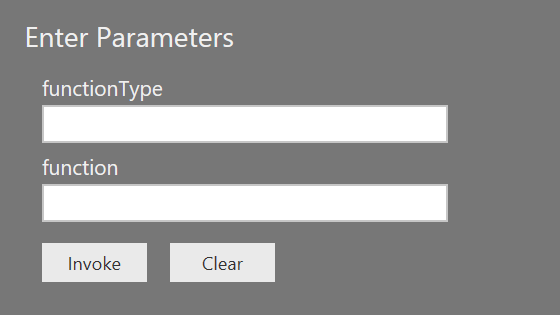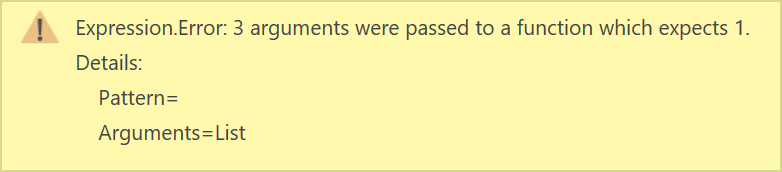# New M function: Function.FromThe November 2017 release of Power BI Desktop contains a new M function: Function.From. Here is the function’s description from the official documentation:

Takes a unary function function and creates a new function with the type functionType that constructs a list out of its arguments and passes it to function.

A unary function is a function that takes one argument. In this case it means that all your arguments will be combined into one list, which will then be the unary function’s argument.

### Examples

Here is the first documented example:

``````// Usage:
Function.From (
type function ( a as number, b as number ) as number,
List.Sum
) ( 2, 1 )
// Output: 3``````

As you can see, the arguments — 2 and 1 — are combined into a list, and then List.Sum works on this list, resulting in 3.

Even though there are only two parameters — a and b — declared in function type, you can invoke the function with as many arguments as you want. The following code works as well:

``````// Usage:
Function.From (
type function ( a as number, b as number ) as number,
List.Sum
) ( 1, 2, 3, 4, 5, 6, 7, 8, 9, 10 )
// Output: 55``````

The fact that your arguments are merged into a single list does not prevent you from creating more sophisticated functions. Here is the second documented example:

``````// Usage:
Function.From (
type function ( a as text, b as text ) as text,
( list ) => list { 0 } & list { 1 }
) ( "2", "1" )
// Output: "21"``````

This example shows that you can create custom functions as usual, except you need to reference list values instead of parameter names. Note that it does not matter what the parameters are called in functionType, because they are all combined in a list anyway.

### Use cases

In a way, the new function acts as a wrapper for Value.ReplaceType when you work with functions. Previously, if you wanted to add some metadata to your function, such as name or description, you had to use Value.ReplaceType, like so:

``````// fOldWay
Value.ReplaceType (
( x ) => x + 1,
type function ( x as number ) as number meta [
Documentation.Description = "This function adds one."
]
)``````

``````// fNewWay
Function.From (
type function ( x as number ) as number meta [
Documentation.Description = "This function adds one."
],
( list ) => list { 0 } + 1
)``````

Apart from improved readability, Function.From has another side effect. Because it combines all of its arguments in a list, you can safely call fNewWay with more than one argument — all arguments but the first one will simply be ignored. Calling fOldWay with three arguments will result in the following error:This behavior should be taken into account if you try to catch errors.